Question

# Consider the truss If the roller at B can sustain a maximum load of 3KN. determine the largest magnitude of each of the three forces F that can be Supported by the truss.

Consider the truss If the roller at B can sustain a maximum load of 3KN. determine the largest magnitude of each of the three forces F that can be Supported by the truss.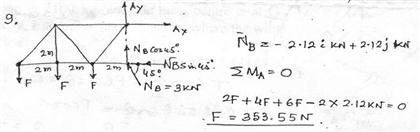#### Earn Coins

Coins can be redeemed for fabulous gifts.

Similar Homework Help Questions
• ### The truss is constructed from three aluminum alloy members, each having a cross-sectional area of A...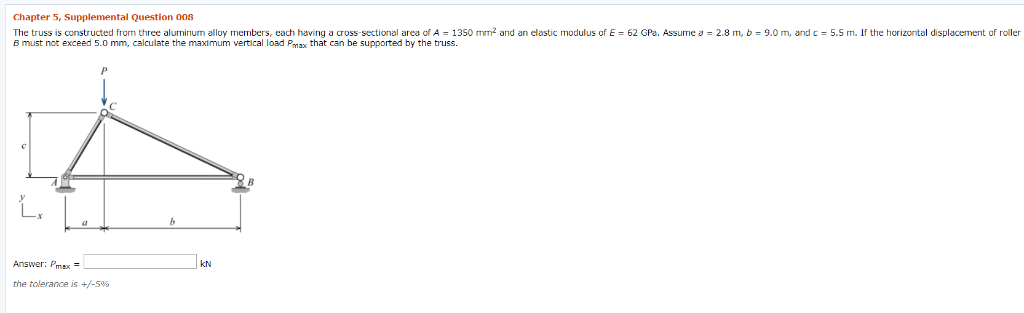The truss is constructed from three aluminum alloy members, each having a cross-sectional area of A = 1350 mm2 and an elastic modulus of E = 62 GPa. Assume a = 2.8 m, b = 9.0 m, and c = 5.5 m. If the horizontal displacement of roller B must not exceed 5.0 mm, calculate the maximum vertical load Pmax that can be supported by the truss. Chapter 5, Supplemental Question 008 The truss is constructed from three aluminum alloy...

• ### The structure below is supported by a pin at A and a roller at E Determine the force in each memb...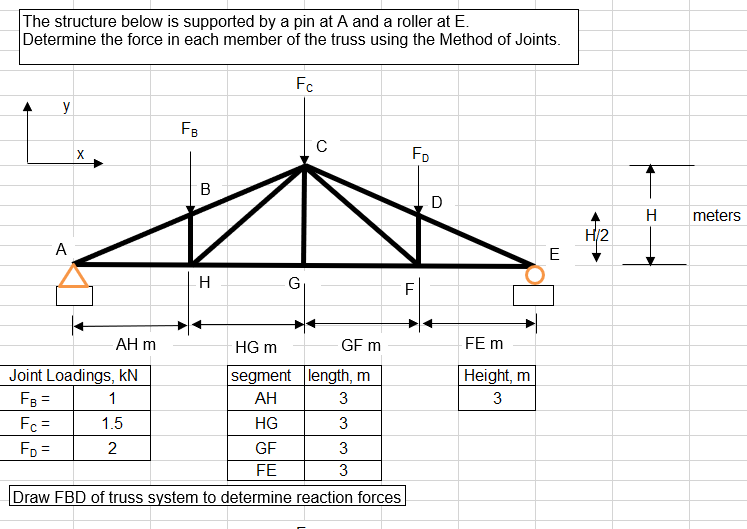The structure below is supported by a pin at A and a roller at E Determine the force in each member of the truss using the Method of Joints H meters 2 FE m AH m GF m HG m Joint Loadings, kN ent length, m Height, m HG GF FE 1.5 2 Draw FBD of truss system to determine reaction forces Assume all forces in members are tensile. If answer is negative, force is compressive. s in x direction...

• ### The truss is constructed from three aluminum alloy members, each having a cross- sectional area of...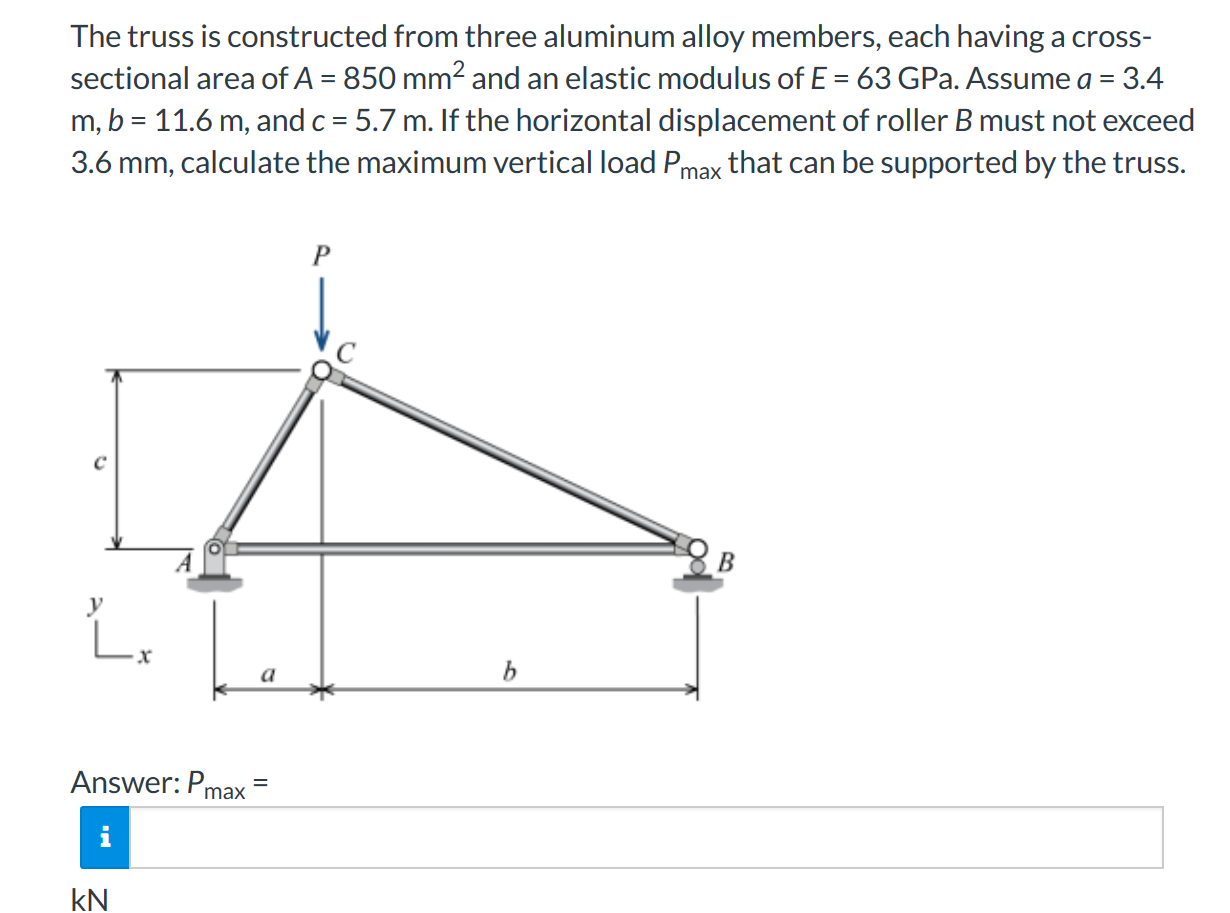The truss is constructed from three aluminum alloy members, each having a cross- sectional area of A = 850 mm2 and an elastic modulus of E = 63 GPa. Assume a = 3.4 m, b = 11.6 m, and c = 5.7 m. If the horizontal displacement of roller B must not exceed 3.6 mm, calculate the maximum vertical load Pmax that can be supported by the truss. Answer: Pmax = KN

• ### Part A - Determining the forces in three specified members of the truss Determine the forces...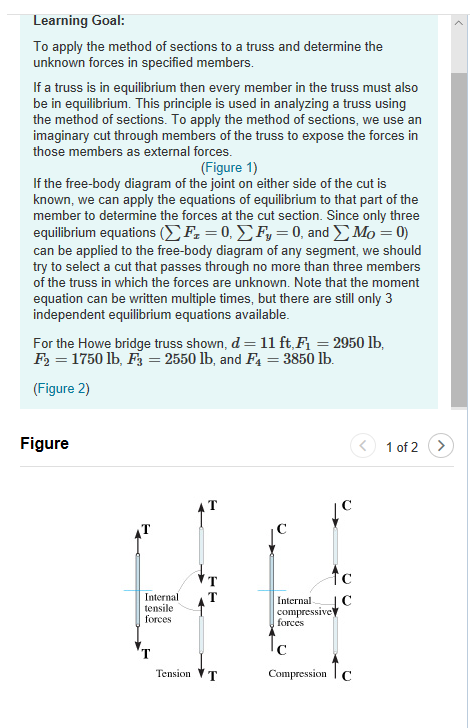Part A - Determining the forces in three specified members of the truss Determine the forces in members CD, DH, and GH. Express the magnitudes of the forces in pounds to three significant figures. Separate your answers with commas. Part B - The Sense of the Forces State if the members considered in part A are in tension or compression. Complete each sentenc e by dragging the appropriate word into the blank. The words can be used more than once....

• ### Review The truss is supported by a pin at A and a roller at B. (Figure...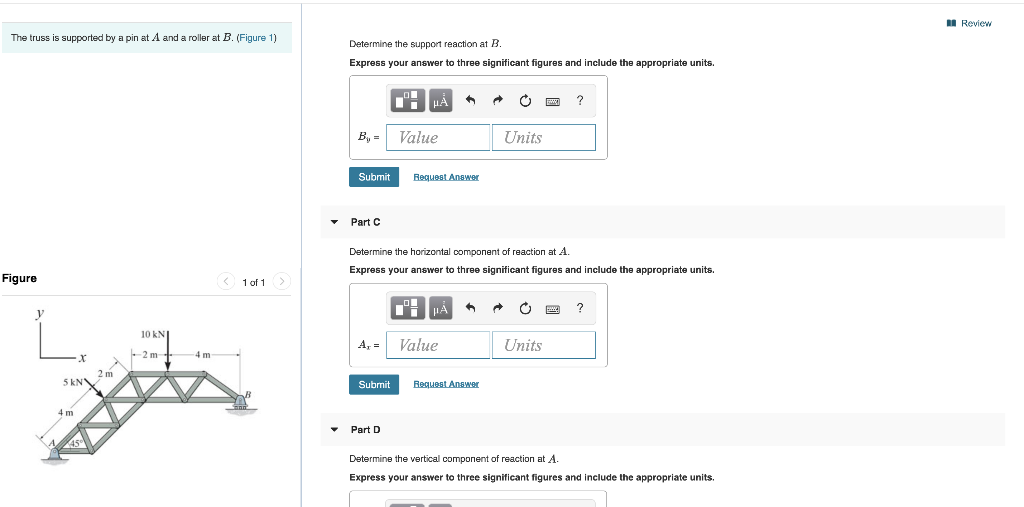Review The truss is supported by a pin at A and a roller at B. (Figure 1) Determine the support reaction at B. Express your answer to three significant figures and include the appropriate units. LE THẢ * * 0 Ea ? B, - Value Units Submit Request Answer Part C Determine the horizontal component of reaction at A. Express your answer to three significant figures and include the appropriate units. Figure 1 of 1 ? CHA Value O Units...

• ### The three-member truss is used to support the vertical load P. Determine the angle θ that satisfies the following conditions simultaneously: 1. The maximum tension force does not exceed 1.25P (12...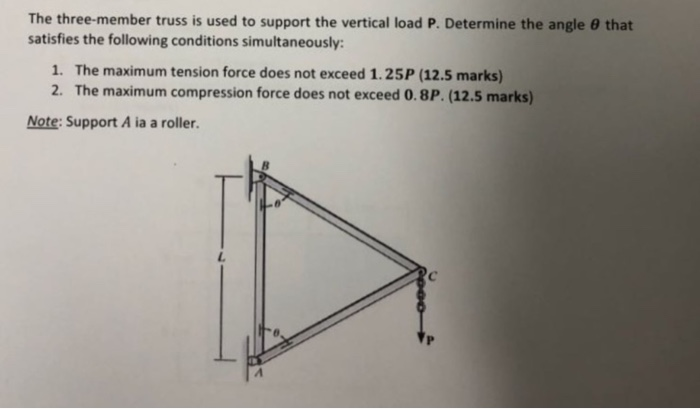The three-member truss is used to support the vertical load P. Determine the angle θ that satisfies the following conditions simultaneously: 1. The maximum tension force does not exceed 1.25P (12.5 marks) 2. The maximum compression force does not exceed 0.8P. (12.5 marks) Note: Support A ia a roller ㄥ The three-member truss is used to support the vertical load P. Determine the angle θ that satisfies the following conditions simultaneously: 1. The maximum tension force does not exceed 1.25P...

• ### (a) Warren truss (b) Howe truss (c) Pratt truss (d) Baltimore truss (e) Parker truss Figure...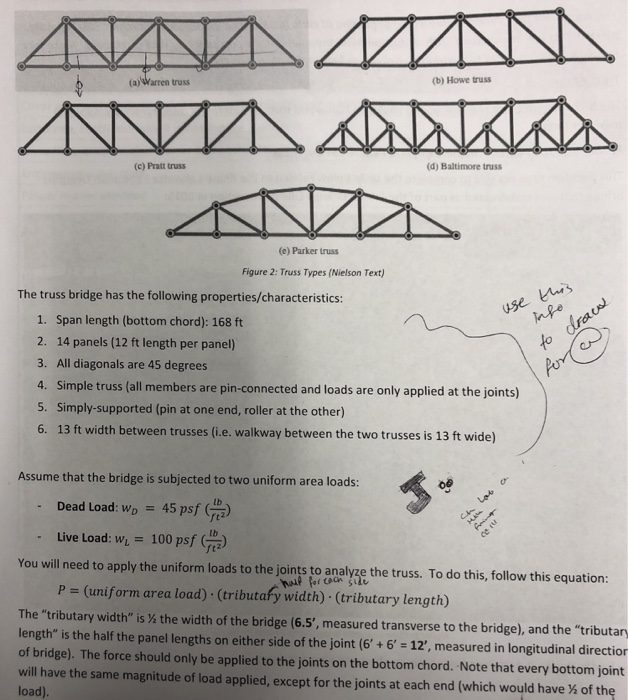(a) Warren truss (b) Howe truss (c) Pratt truss (d) Baltimore truss (e) Parker truss Figure 2: Truss Types (Nielson Text) The truss bridge has the following properties/characteristics: 1. Span length (bottom chord): 168 ft 2. 14 panels (12 ft length per panel) 3. All diagonals are 45 degrees 4. Simple truss (all members are pin-connected and loads are only applied at the joints) 5. Simply-supported (pin at one end, roller at the other) 6. 13 ft width between trusses...

• ### P16.52 Determine the maximum and minimum axial forces in member CH of the truss in Problem 16.45 given the following loading scenario: uniform dead load of 1 klf, a moving uniform live load of 2 klf,...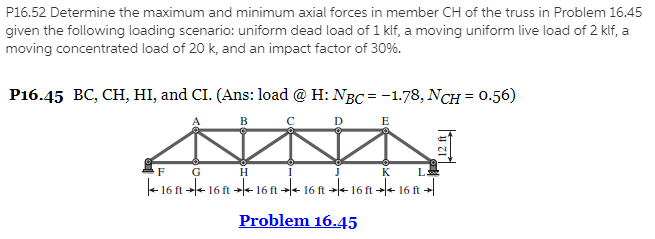P16.52 Determine the maximum and minimum axial forces in member CH of the truss in Problem 16.45 given the following loading scenario: uniform dead load of 1 klf, a moving uniform live load of 2 klf, a moving concentrated load of 20 k, and an impact factor of 30%. P16.45 BC, CH, HI, and CI. (Ans: load @ H: NBC=-1.78, MCH = 0.56) F G Problem 16.45 P16.52 Determine the maximum and minimum axial forces in member CH of the...

• ### KHW#3 Problem 3.34 8 of 15 Part A Earh coed an sustain a maximum tension ofl 25 lb. (Figure 1) Determine the largest we...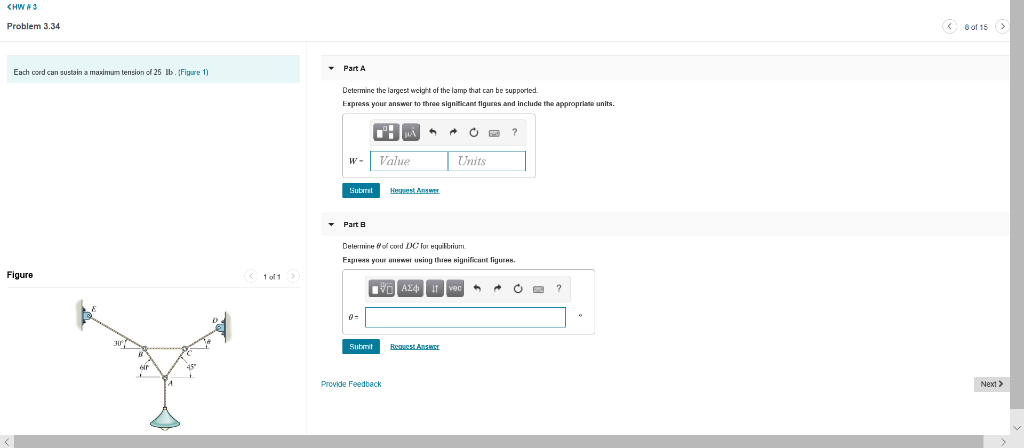KHW#3 Problem 3.34 8 of 15 Part A Earh coed an sustain a maximum tension ofl 25 lb. (Figure 1) Determine the largest weight af the lamp that can be supported. Express vour answer to three significant tiqures and include the appronriate units. ? Units Value и H t Ans Subrit Part B Delemine of ced DC for equlbium Eщpя уон апкин икіп thини ніцniсant fiuннк. Figure 1 ol 1. AEit 0: Bequest AnswI Submit Provide Feedback Next KHW#3 Problem...

• ### Determine the maximum force F that can be supported in the position shown if each chain...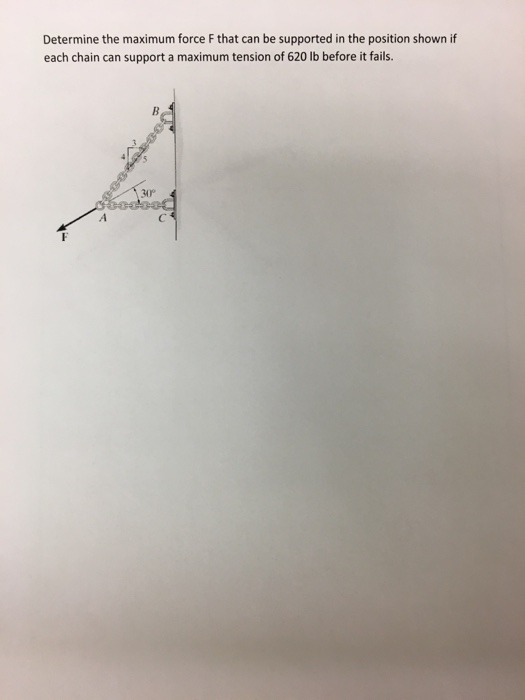Determine the maximum force F that can be supported in the position shown if each chain can support a maximum tension of 620 lb before it fails. 30°